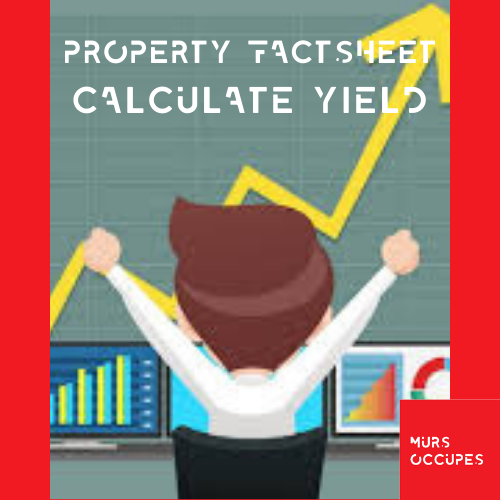# Calculate the profitability

In any investment what matters and what we will expect is profitability. Firstly, the return is essential because it represents the gain that we will receive but also because this calculation makes it possible to compare several returns and therefore constitutes a real decision lever. The return on a property investment is fairly easy to calculate. Indeed, you need certain data such as the monthly payments of your loan, the monthly payments of the future tenants and the future charges. The yield is calculated in gross or net but the net is closer to the real gain that we will make because we add in the calculation the different charges and taxes to which you will be subject. Here are the two formulas to calculate the net and gross yield:
Gross yield:
(Rent amount x 12 months) x 100 / purchase price + purchase costs
Net return:
(Rent amount x 12 months) x 100 - (rental charges) / purchase price + purchase costs